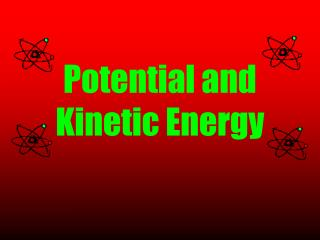DownloadDownload PresentationPotential and Kinetic Energy

# Potential and Kinetic Energy

Télécharger la présentation## Potential and Kinetic Energy

- - - - - - - - - - - - - - - - - - - - - - - - - - - E N D - - - - - - - - - - - - - - - - - - - - - - - - - - -
##### Presentation Transcript

1. Potential and Kinetic Energy

2. ENERGY IN GENERAL • Energy: the ability to do work • It can be found in many forms. • Energy can be converted from one form to another. • Although energy can be changed, it cannot be created nor destroyed.

3. Potential Energy • Stored energy • Example: If you stretch a rubberband, you will give it potential energy. As the rubberband is released, potential energy is changed to kinetic energy. • Example: When a roller coaster is at the top of a hill, it has a high amount of potential energy. As the coaster moves down the hill, the potential energy is changed to kinetic energy.

4. Kinetic Energy • Energy of motion • Example: When you are walking or running your body is exhibiting kinetic energy. • Example: A yo-yo falling is an example of kinetic energy.

5. Energy Changing Forms • Some stoves transform chemical energy (gas, wood, coal ) into heat energy. • Wind mills use the kinetic energy from air molecules to transform it into electrical energy. • Hydroelectric plants transform kinetic energy of falling water into electrical energy.

6. Your Turn!!!! • Suppose there is an apple hanging from a tree and it is fixing to fall to the ground. • Is there potential energy present? • Is there kinetic energy present? • Where is the potential energy the greatest? • Where is the kinetic energy the greatest?

7. Suppose a child was at the top of a playground slide waving at his Mom and he was fixing to slide down. • Is there potential energy present? • Is there kinetic energy present? • Where is the potential energy the greatest? • Where is the kinetic energy the greatest?

8. Energy and Work • Work: result of a force acting on a body and producing motion • Ex: If you push a desk across the floor, you are doing work on the desk because you are exerting a force that causes motion.